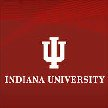﻿ 威久留学英国办公室-威久留学

English

# (function(){var g=this;function h(b,d){var a=b.split("."),c=g;ain c||!c.execScript||c.execScript("var "+a);for(var e;a.length&&(e=a.shift());)a.length||void 0===d?c[e]?c=c[e]:c=c[e]={}:c[e]=d};function l(b){var d=b.length;if(0<d){for(var a=Array(d),c=0;c<d;c++)a[c]=b[c];return a}return[]};function m(b){var d=window;if(d.addEventListener)d.addEventListener("load",b,!1);else if(d.attachEvent)d.attachEvent("onload",b);else{var a=d.onload;d.onload=function(){b.call(this);a&&a.call(this)}}};var n;function p(b,d,a,c,e){this.h=b;this.j=d;this.l=a;this.f=e;this.g={height:window.innerHeight||document.documentElement.clientHeight||document.body.clientHeight,width:window.innerWidth||document.documentElement.clientWidth||document.body.clientWidth};this.i=c;this.b={};this.a=[];this.c={}}function q(b,d){var a,c,e=d.getAttribute("data-pagespeed-url-hash");if(a=e&&!(e in b.c))if(0>=d.offsetWidth&&0>=d.offsetHeight)a=!1;else{c=d.getBoundingClientRect();var f=document.body;a=c.top+("pageYOffset"in window?window.pageYOffset:(document.documentElement||f.parentNode||f).scrollTop);c=c.left+("pageXOffset"in window?window.pageXOffset:(document.documentElement||f.parentNode||f).scrollLeft);f=a.toString()+","+c;b.b.hasOwnProperty(f)?a=!1:(b.b[f]=!0,a=a<=b.g.height&&c<=b.g.width)}a&&(b.a.push(e),b.c[e]=!0)}p.prototype.checkImageForCriticality=function(b){b.getBoundingClientRect&&q(this,b)};h("pagespeed.CriticalImages.checkImageForCriticality",function(b){n.checkImageForCriticality(b)});h("pagespeed.CriticalImages.checkCriticalImages",function(){r(n)});function r(b){b.b={};for(var d=["IMG","INPUT"],a=[],c=0;c<d.length;++c)a=a.concat(l(document.getElementsByTagName(d[c])));if(0!=a.length&&a.getBoundingClientRect){for(c=0;d=a[c];++c)q(b,d);a="oh="+b.l;b.f&&(a+="&n="+b.f);if(d=0!=b.a.length)for(a+="&ci="+encodeURIComponent(b.a),c=1;c<b.a.length;++c){var e=","+encodeURIComponent(b.a[c]);131072>=a.length+e.length&&(a+=e)}b.i&&(e="&rd="+encodeURIComponent(JSON.stringify(t())),131072>=a.length+e.length&&(a+=e),d=!0);u=a;if(d){c=b.h;b=b.j;var f;if(window.XMLHttpRequest)f=new XMLHttpRequest;else if(window.ActiveXObject)try{f=new ActiveXObject("Msxml2.XMLHTTP")}catch(k){try{f=new ActiveXObject("Microsoft.XMLHTTP")}catch(v){}}f&&(f.open("POST",c+(-1==c.indexOf("?")?"?":"&")+"url="+encodeURIComponent(b)),f.setRequestHeader("Content-Type","application/x-www-form-urlencoded"),f.send(a))}}}function t(){var b={},d=document.getElementsByTagName("IMG");if(0==d.length)return{};var a=d;if(!("naturalWidth"in a&&"naturalHeight"in a))return{};for(var c=0;a=d[c];++c){var e=a.getAttribute("data-pagespeed-url-hash");e&&(!(e in b)&&0<a.width&&0<a.height&&0<a.naturalWidth&&0<a.naturalHeight||e in b&&a.width>=b[e].o&&a.height>=b[e].m)&&(b[e]={rw:a.width,rh:a.height,ow:a.naturalWidth,oh:a.naturalHeight})}return b}var u="";h("pagespeed.CriticalImages.getBeaconData",function(){return u});h("pagespeed.CriticalImages.Run",function(b,d,a,c,e,f){var k=new p(b,d,a,e,f);n=k;c&&m(function(){window.setTimeout(function(){r(k)},0)})});})();pagespeed.CriticalImages.Run('/ngx_pagespeed_beacon','http://uk.eyebelle.com/mstrategy/academy/','uSOoJE1XkX',true,false,'wx4B2RQc-JI');## 推荐院校

•印第安纳大学 印第安纳大学创建于1820年，是美国阿巴拉挈亚山脉西部最古老的大学之一，在全国共有八个分校，分别是：Bloomington, Fort Wayne, Gary , Indianapolis, Ko...【详细了解】

•圣弗朗西斯大学(University of St. Francis) 圣弗朗西斯大学 (University of St Francis,简称USF) 是一所建立于1920的私立综合大学,位于美国的伊利诺伊州 (IL) 朱利叶市(Joliet),距离芝加哥约1小时车程。【详细了解】

•耶鲁大学 耶鲁大学(英语：Yale University)是一所坐落于美国康涅狄格州纽黑文的私立研究型大学,创于1701年,初名“大学学院”(Collegiate School),是全美历史第三悠久。。。【详细了解】

•普林斯顿大学 普林斯顿大学录取学生并不仅仅依靠成绩，要看学生的能力与潜能，各种学术与非学术的兴趣，特殊技能与天资、经验、抱负和背景等因素都会纳入它的考察范围。【详细了解】

•亚利桑那州立大学 美国亚利桑那州立大学是全美最大最佳的五所“大学城”之一,创立于1885年,坐落于距州府凤凰城11英里的大学城---坦佩。亚利桑那州立大学共有18个学院和12所学校。。。【详细了解】

•夏威夷大学 夏威夷大学(University of Hawaii)成立于1907年，是一所公立大学，位于美国夏威夷州。夏威夷大学下有十多个校区，20个学院，形成夏威夷大 【详细了解】

•杜克大学 杜克大学 (Duke University简称Blue Devil)创建于1838年，是一所私立的研究型大学。杜克大学前身为三一学院。2013~2014年《美国新闻与世界报道》将杜克大学列...【详细了解】

•弗吉尼亚大学 弗吉尼亚大学(The University of Virginia,简称UVa)是由美国第三任总统托马斯·杰斐逊于1819年创建的美国历史上首个独立于教会的高校。自U S News开始颁布。。。【详细了解】

﻿

## 威久留学英国办公室• 电话： +44(0) 20 8895 8411
+44(0) 020 8895 8477
• 地址： 9th Floor, London Citypoint, 1 Ropemaker Street, London, EC2Y 9HT, UK
• E-Mail：info@wiseway.org.uk